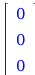Boltzmann - Maple Help

Boltzmann DistributionOverview In quantum mechanics the energies of atoms and molecules, whether they be electronic, vibrational, or rotational, are discrete or quantized.   The Boltzmann distribution, developed by Ludwig Boltzmann, gives the probability of finding a quantum atom or molecule in a given energy state as a function of the temperature.  In this lesson we will study the Boltzmann distribution for vibrational energies of the diatomic molecule hydrogen chloride.Figure 1: Boltzmann distribution for the vibrational energy states of hydrogen chloride at 8409 K.Boltzmann Distribution The Boltzmann distribution is where Pn(T) is the probability of finding the atom or molecule in the nth quantum state, En is the energy of the quantum state, T is the temperature, and k is the Boltzmann constant.  The denominator of the expression is just a normalization to make the sum of all probabilities Pn(T) equal one.Diatomic MoleculeVibrational Energies

In quantum mechanics the vibrational energies of a diatomic molecule can be approximated by a harmonic spring (oscillator) potential

${E}_{{n}}=h\cdot \mathrm{ν}\cdot \left(n+\frac{1}{2}\right)$

where n is an integer quantum number ranging from 0 to ∞, ν is the frequency of the spring and h is Planck's constant.

The lowest vibrational energy can be computed with the Quantum Chemistry package.  Let us set the Digits to 15 and load the package

 >
 $\left[{\mathrm{AOLabels}}{,}{\mathrm{ActiveSpaceCI}}{,}{\mathrm{ActiveSpaceSCF}}{,}{\mathrm{AtomicData}}{,}{\mathrm{BondAngles}}{,}{\mathrm{BondDistances}}{,}{\mathrm{Charges}}{,}{\mathrm{ChargesPlot}}{,}{\mathrm{CorrelationEnergy}}{,}{\mathrm{CoupledCluster}}{,}{\mathrm{DensityFunctional}}{,}{\mathrm{DensityPlot3D}}{,}{\mathrm{Dipole}}{,}{\mathrm{DipolePlot}}{,}{\mathrm{Energy}}{,}{\mathrm{ExcitationEnergies}}{,}{\mathrm{ExcitationSpectra}}{,}{\mathrm{ExcitationSpectraPlot}}{,}{\mathrm{ExcitedStateEnergies}}{,}{\mathrm{ExcitedStateSpins}}{,}{\mathrm{FullCI}}{,}{\mathrm{GeometryOptimization}}{,}{\mathrm{HartreeFock}}{,}{\mathrm{Interactive}}{,}{\mathrm{Isotopes}}{,}{\mathrm{MOCoefficients}}{,}{\mathrm{MODiagram}}{,}{\mathrm{MOEnergies}}{,}{\mathrm{MOIntegrals}}{,}{\mathrm{MOOccupations}}{,}{\mathrm{MOOccupationsPlot}}{,}{\mathrm{MOSymmetries}}{,}{\mathrm{MP2}}{,}{\mathrm{MolecularData}}{,}{\mathrm{MolecularGeometry}}{,}{\mathrm{NuclearEnergy}}{,}{\mathrm{NuclearGradient}}{,}{\mathrm{OscillatorStrengths}}{,}{\mathrm{Parametric2RDM}}{,}{\mathrm{PlotMolecule}}{,}{\mathrm{Populations}}{,}{\mathrm{RDM1}}{,}{\mathrm{RDM2}}{,}{\mathrm{RTM1}}{,}{\mathrm{ReadXYZ}}{,}{\mathrm{Restore}}{,}{\mathrm{Save}}{,}{\mathrm{SaveXYZ}}{,}{\mathrm{SearchBasisSets}}{,}{\mathrm{SearchFunctionals}}{,}{\mathrm{SkeletalStructure}}{,}{\mathrm{Thermodynamics}}{,}{\mathrm{TransitionDipolePlot}}{,}{\mathrm{TransitionDipoles}}{,}{\mathrm{TransitionOrbitalPlot}}{,}{\mathrm{TransitionOrbitals}}{,}{\mathrm{Variational2RDM}}{,}{\mathrm{VibrationalModeAnimation}}{,}{\mathrm{VibrationalModes}}{,}{\mathrm{Video}}\right]$ (3.1.1)

Define the molecule hydrogen chloride at its equilibrium geometry

 >
 ${\mathrm{mol}}{≔}\left[\left[{"H"}{,}{0}{,}{0}{,}{0}\right]{,}\left[{"Cl"}{,}{1.31300000}{,}{0}{,}{0}\right]\right]$ (3.1.2)

We can compute the lowest vibrational mode in cm-1 with the command VibrationalModes (may take a minute)

 >
 ${\mathrm{mode}}{≔}{3374.82528900}{}⟦\frac{{1}}{{\mathrm{cm}}}⟧$ (3.1.3)

The vibration of hydrogen chloride in its ground vibrational state can be animated with the command VibrationalModeAnimation.  After executing the command, click on the plot and click play to see one vibration (note when you click on the plot, a plot menu appears; by changing with the menu icon to the left of fps, you can make the vibrations run continuously until you press pause or stop!)

 > $\mathrm{VibrationalModeAnimation}\left(\mathrm{mol},\mathrm{modes},\mathrm{vecs},1\right);$We can convert cm-1 (cycles per cm) to Hz (cycles per s) by multiplying by the speed of light c

 >
 ${{c}}_{{\mathrm{SI}}}{≔}{2.99792458}{}{{10}}^{{8}}{}⟦\frac{{m}}{{s}}⟧$ (3.1.4)
 >
 ${\mathrm{\nu }}{≔}{1.01174717}{}{{10}}^{{12}}{}⟦\frac{{1}}{{\mathrm{cm}}}⟧{}⟦\frac{{m}}{{s}}⟧$ (3.1.5)

or combining units with Maple

 >
 ${\mathrm{\nu }}{≔}{1.01174717}{}{{10}}^{{14}}{}⟦\frac{{1}}{{s}}⟧$ (3.1.6)

To obtain the vibrational energies, we need Planck's constant

 >
 ${{h}}_{{\mathrm{SI}}}{≔}{6.62607004}{}{{10}}^{{-34}}{}⟦\frac{{{m}}^{{2}}{}{\mathrm{kg}}}{{s}}⟧$ (3.1.7)

Let us define the vibrational energies of hydrogen chloride as a function of the quantum number n

 >
 ${E}{≔}{n}{↦}{{h}}_{{\mathrm{SI}}}{\cdot }{\mathrm{\nu }}{\cdot }\left({n}{+}\frac{{1}}{{2}}\right)$ (3.1.8)

With the function we can compute the first 10 vibrational energy states in Joules

 >
 ${\mathrm{energies}}{≔}\left[{3.35195380}{}{{10}}^{{-20}}{}⟦{J}⟧{,}{1.00558614}{}{{10}}^{{-19}}{}⟦{J}⟧{,}{1.67597690}{}{{10}}^{{-19}}{}⟦{J}⟧{,}{2.34636766}{}{{10}}^{{-19}}{}⟦{J}⟧{,}{3.01675842}{}{{10}}^{{-19}}{}⟦{J}⟧{,}{3.68714918}{}{{10}}^{{-19}}{}⟦{J}⟧{,}{4.35753994}{}{{10}}^{{-19}}{}⟦{J}⟧{,}{5.02793070}{}{{10}}^{{-19}}{}⟦{J}⟧{,}{5.69832146}{}{{10}}^{{-19}}{}⟦{J}⟧{,}{6.36871222}{}{{10}}^{{-19}}{}⟦{J}⟧\right]$ (3.1.9)
 > 

(a) What is the frequency of the hydrogen chloride bond in Hz?

(b) What are the energies of the three lowest vibrational energies?

In the next section we will use the Boltzmann distribution to determine the probability of a hydrogen chloride molecule being in a given vibrational energy state.Boltzmann Distribution

To evaluate the Boltzmann distribution, we need he Boltzmann constant k

 >
 ${{k}}_{{\mathrm{SI}}}{≔}{1.38064851}{}{{10}}^{{-23}}{}⟦\frac{{{m}}^{{2}}{}{\mathrm{kg}}}{{{s}}^{{2}}{}{K}}⟧$ (3.2.1)

Let us define a Maple procedure to evaluate the Boltzmann distribution for an input list of energies

 >
 ${P}{≔}{\mathbf{proc}}\left({\mathrm{energies}}{,}{T}\right)\phantom{\rule[-0.0ex]{0.5em}{0.0ex}}{\mathbf{local}}\phantom{\rule[-0.0ex]{0.5em}{0.0ex}}{\mathrm{norm}}{,}{e}{;}\phantom{\rule[-0.0ex]{0.5em}{0.0ex}}{\mathrm{norm}}{≔}{\mathrm{add}}{}\left({\mathrm{exp}}{}\left({\mathrm{combine}}{}\left({−}{e}{/}\left({k}{[}{\mathrm{SI}}{]}{*}{T}\right){,}{\mathrm{units}}\right)\right){,}{e}\phantom{\rule[-0.0ex]{0.5em}{0.0ex}}{\mathbf{in}}\phantom{\rule[-0.0ex]{0.5em}{0.0ex}}{\mathrm{energies}}\right){;}\phantom{\rule[-0.0ex]{0.5em}{0.0ex}}\left[{\mathrm{seq}}{}\left({\mathrm{exp}}{}\left({\mathrm{combine}}{}\left({−}{e}{/}\left({k}{[}{\mathrm{SI}}{]}{*}{T}\right){,}{\mathrm{units}}\right)\right){/}{\mathrm{norm}}{,}{e}\phantom{\rule[-0.0ex]{0.5em}{0.0ex}}{\mathbf{in}}\phantom{\rule[-0.0ex]{0.5em}{0.0ex}}{\mathrm{energies}}\right)\right]\phantom{\rule[-0.0ex]{0.5em}{0.0ex}}{\mathbf{end proc}}$ (3.2.2)

For T = 298 K what are the probabilities for the molecule being in each of the vibrational energy states?

 >
 ${\mathrm{probabilities}}{≔}\left[{0.99999992}{,}{8.38669326}{}{{10}}^{{-8}}{,}{7.03366298}{}{{10}}^{{-15}}{,}{5.89891789}{}{{10}}^{{-22}}{,}{4.94724191}{}{{10}}^{{-29}}{,}{4.14910039}{}{{10}}^{{-36}}{,}{3.47972352}{}{{10}}^{{-43}}{,}{2.91833763}{}{{10}}^{{-50}}{,}{2.44752046}{}{{10}}^{{-57}}{,}{2.05266050}{}{{10}}^{{-64}}\right]$ (3.2.3)

(c) What is the probability of a hydrogen chloride molecule being in its lowest (n = 0) vibrational energy state at 298 K?

We can plot the probabilities in a bar graph as a function of temperature.  Using the slide rule, change the temperature to explore how the Boltzmann distribution changes with increasing temperature.

 >

$\mathbf{T}$> 

(d) Using the interactive plot, estimate the probability of a hydrogen chloride molecule being in its lowest (n = 0) vibrational energy state at approximately 5000 K?

(e) Using the interactive plot, estimate the probability of a hydrogen chloride molecule being in its first excited (n = 1) vibrational energy state at approximately 5000 K?

(f) In a gas of 1000 hydrogen chloride molecules, approximately how many would you expect to be in the second excited (n = 2) vibrational energy state at about 5000 K?